Simulation

Simulation

Mechanics

mechanics
- statics             靜力學：有力，呈穩態（處處總和為零）
- kinematics          運動學：無力
- dynamics = kinects  動力學：有力

System

system
- structure   結構系統：不動
- mechanism   機械系統：會動

UVa 11574

Particle Simulation

Particle Simulation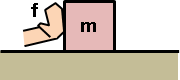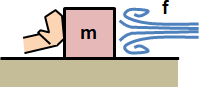http://allenchou.net/2015/04/game-math-precise-control-over-numeric-springing/

Fluid Simulation

Fluid Simulationhttp://bugman123.com/FluidMotion/
http://www.mijagourlay.com/fluid
https://www.cs.clemson.edu/savage/pba/
https://fluidenginedevelopment.org/
https://developer.nvidia.com/
http://pof.tnw.utwente.nl/research
http://panoramx.ift.uni.wroc.pl/~maq/eng/index.php

Fluid Simulation (Particle)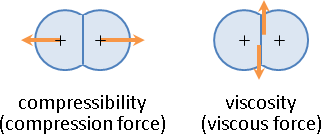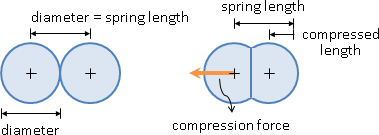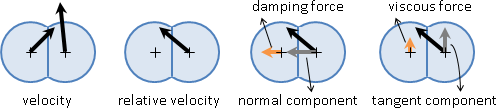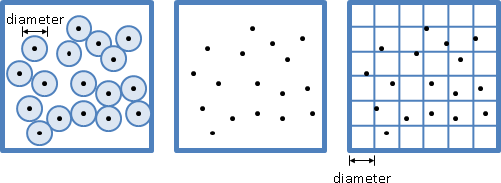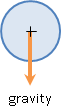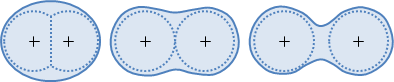Fluid Simulation (Grid)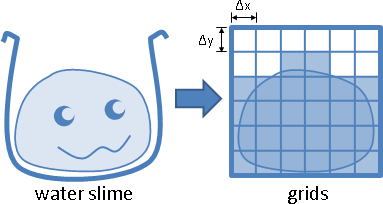colocated grid：速度在格中央。
staggered grid：X速度在格左右，Y速度在格上下。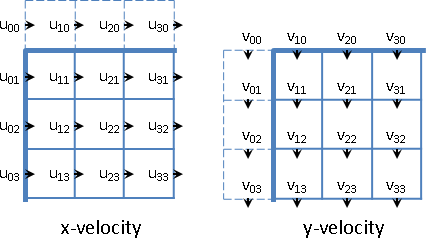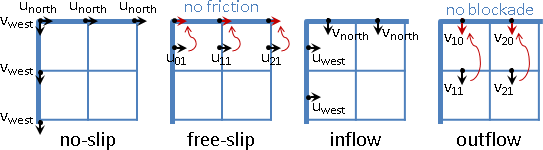no-slip condition：牆壁沾黏，切線速度是特定值。
free-slip condition：牆壁光滑，切線速度等於鄰水速度。
inflow condition：牆壁插管，法線速度是特定值。
outflow condition：牆壁虛設，法線速度等於鄰水速度。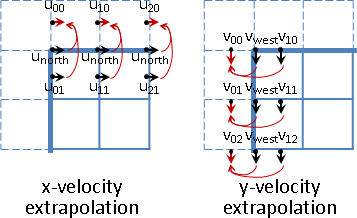diffuse：擴散。格中粒子均勻四散，其速度也隨之均勻四散。

∂          ∂       ∂         ∂          ∂       ∂
―― u = ν ( ――― u + ――― u )   ―― v = ν ( ――― v + ――― v )
∂t         ∂x²     ∂y²    ,  ∂t         ∂x²     ∂y²
^^^                          ^^^
這兩個是擴散速率。希臘字母ν，唸做/nju:/，寫做nu，不是英文字母v。

flow：流動。格中粒子依照自身速度集體移動，其速度也隨之集體移動。

∂          ∂       ∂         ∂          ∂       ∂
―― u = - ( ―― uu + ―― vu )   ―― v = - ( ―― uv + ―― vv )
∂t         ∂x      ∂y     ,  ∂t         ∂x      ∂yincompressibility：不可壓縮。

∂      ∂
―― u + ―― v = 0
∂x     ∂y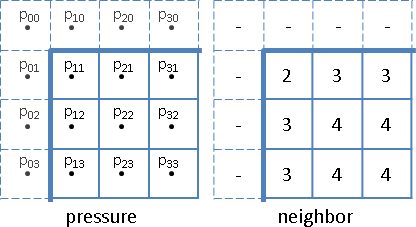SOR：稀疏矩陣，適合鬆弛法。容易實作，但是收斂速度慢。
PCG：半正定矩陣，適合共軛梯度法。請見這篇講義one-step method
1. 抓本地速度，走一步。

predictor-corrector method
1. 抓本地速度，走一步，到終點。
2. 抓本地與終點的平均速度，從頭重新走一步。

midpoint method
1. 抓本地速度，走半步，到中點。
2. 抓中點速度，從頭重新走一步。forward

backward

牆壁事先設定粒子數量，方便內插。

projection method
1. flow，走一步。V1 = V0 + V0 ⋅ Δt
2. project，減掉散場。V1p = V1 - div_part(V1)

reflection method [Zehnder et al 2018]
1. flow，走半步。V½ = V0 + V0 ⋅ ½Δt
2. project，減掉散場。V½p = V½ - div_part(V½)
3. reflect，再減掉一次散場。V½r = V½ - div_part(V½) - div_part(V½)
4. flow，走半步。V1 = V½r + V½p ⋅ ½Δt
5. project，減掉散場。V1p = V1 - div_part(V1)

modified MacCormack method
1. 更新速度場。V0 -> V1
2. 速度變號，再更新速度場。V1 -> V2
理論上回復原狀：V0 = V2
實際上產生誤差：V0 ≠ V2
3. 來回更新兩次，因此更新一次的誤差是一半：(V2 - V0) / 2
4. 正確答案是更新一次，再扣掉更新一次的誤差：V1 - (V2 - V0) / 2
5. 裁切答案，不超過V1四周的最大值和最小值。

marker particles

front tracking

volume of fluid

level set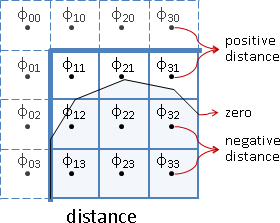八鄰居取距離最小者，只取已有距離的格子。八鄰居的速度平均數，只取已有速度的格子。Continuity Equation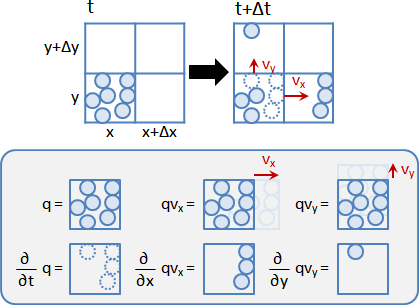∂
―― q                             ---> q̇
∂t

∂       ∂
―― qu + ―― qv                    ---> div(qu)
∂x      ∂y

∂        ∂       ∂
―― q + ( ―― qu + ―― qv ) = 0     ---> q̇ + div(qu) = 0
∂t       ∂x      ∂y
^^^^   ^^^^^^^^^^^^^^^^^
time   space difference∂          ∂        ∂            ∂      ∂
―― q + ( u ―― q + v ―― q ) + q ( ―― u + ―― v ) = 0
∂t         ∂x       ∂y           ∂x     ∂y
^^^^^^^^^^^^^^^^^^^     ^^^^^^^^^^^^^^^

∂        ∂
( u ―― q + v ―― q )              ---> u dot grad(q)
∂x       ∂y

∂      ∂
q ( ―― u + ―― v )                ---> q div(u)
∂x     ∂y

∂      ∂
q ( ―― u + ―― v ) = 0            ---> q div(u) = 0
∂x     ∂y

∂          ∂        ∂
―― q + ( u ―― q + v ―― q ) = 0   ---> q̇ + (u dot grad(q)) = 0
∂t         ∂x       ∂y
^^^^   ^^^^^^^^^^^^^^^^^^^

D
―― q = 0
Dt

Navier–Stokes Equation

∂        ∂       ∂
―― ρ + ( ―― ρu + ―― ρv ) = 0
∂t       ∂x      ∂y

{  ∂         ∂        ∂            ∂        ∂        ∂
{  ―― ρu + ( ―― ρuu + ―― ρvu ) = - ―― p + ( ―― τ   + ―― τ   )
{  ∂t        ∂x       ∂y           ∂x       ∂x  ˣˣ   ∂y  ˣʸ
{
{  ∂         ∂        ∂            ∂        ∂        ∂
{  ―― ρv + ( ―― ρuv + ―― ρvv ) = - ―― p + ( ―― τ   + ―― τ   )
{  ∂t        ∂x       ∂y           ∂x       ∂x  ʸˣ   ∂y  ʸʸ
^^^^^   ^^^^^^^^^^^^^^^^^^^     ^^^^   ^^^^^^^^^^^^^^^^^^^
time          space            intra         inter
difference     difference         force         force

Navier–Stokes Equation for Water

∂        ∂
―― τ   + ―― τ
∂x  ˣˣ   ∂y  ˣʸ

∂      ∂        ∂      ∂
= ―― ( μ ―― u ) + ―― ( μ ―― u )
∂x     ∂x       ∂y     ∂y

∂       ∂
= μ ( ――― u + ――― u )       ---> μ laplace(u)
∂x²     ∂y²

∂        ∂
―― ρuu + ―― ρvu
∂x       ∂y
^^^^^^^^^^^^^^^
space difference

∂         ∂              ∂      ∂
= ( u ―― ρu + v ―― ρu ) + ρu ( ―― u + ―― v )
∂x        ∂y             ∂x     ∂y
^^^^^^^^^^^^^^^^^^^^^      ^^^^^^^^^^^^^^^

∂         ∂
= ( u ―― ρu + v ―― ρu )     ---> u dot grad(ρu)
∂x        ∂y

∂           ∂         ∂
―― ρu + ( u ―― ρu + v ―― ρu )
∂t          ∂x        ∂y

∂          ∂        ∂
= ρ [ ―― u + ( u ―― u + v ―― u ) ]     ---> ρ [ u̇ + (u dot grad(u)) ]
∂t         ∂x       ∂y

{ ρ [ u̇ + (u dot grad(u)) ] = - grad(p) + μ laplace(u)
{ div(u) = 0
{ ρ ( u̇ + u·∇u ) = -∇p + μ ∆u
{ ∇·u = 0
{ u̇ + u·∇u = -∇p/ρ + ν ∆u     (let μ/ρ = ν)
{ ∇·u = 0

Navier–Stokes Equation for 2D Water

pressure-velocity formulation
{ u̇ + (u dot grad(u)) = -(grad(p) / ρ) + ν laplace(u)
{ div(u) = 0

streamfunction-vorticity formulation
{ laplace(ψ) = -ω

Schrödinger Equation for Water

Solid Simulation（Under Construction!）

Solid Simulation

n-body       https://en.wikipedia.org/wiki/Barnes–Hut_simulation
cloth        http://www.cs.cornell.edu/projects/YarnCloth/
hair         http://www.andyselle.com/papers/9/
soft object  http://www.alecrivers.com/fastlsm/
water wave   http://www.cemyuksel.com/research/waveparticles/
matter       http://chemists.princeton.edu/torquato/Structural Optimization: Topology Optimization。調整模型結構。
Molecular Modeling: Energy Minimization。調整化學結構。

Collision

http://box2d.org/
https://www.atmos.illinois.edu/courses/atmos100/userdocs/3Dcollisions.html
http://en.wikipedia.org/wiki/N-body_problem

Fracture

Strand

Cloth

Water Wave

Deformable Simulation（Under Construction!）

Deformation

http://en.wikipedia.org/wiki/Soft_body_dynamics
https://www.seas.upenn.edu/~cffjiang/

《The Linearized Theory of Elasticity》

《Continuum Mechanics Theory》

elastic

http://ddg.cs.columbia.edu/SIGGRAPH05/Shells.pdf
deformation mapping:  x̕ = f(x)       transformation of point
deformation gradient: t̕ = ∇f(t)      transformation of tangent vector
strain energy:        ‖t̕‖² - ‖t‖²    difference of squared length

‖t̕‖² - ‖t‖²
= ‖∇f(t)‖² - ‖t‖²
= tᵀ ∇fᵀ∇f t - tᵀt
= tᵀ (∇fᵀ∇f - I) t
force: F
displacement: ΔL
stress: σ = F/A  ---> df/dx²        area: A
strain: ε = ΔL/L ---> du/dx = ∇ₓu   length: L
stress-strain curve:
hooke: σ = Eε                       young modulus / stiffness tensor: E
hooke: f = kΔL
strain energy:  U = 1/2Vσε = 1/2fΔL
elastic energy: U = 1/2kΔL²
deformation: F(x)
deformation gradient (transformation matrix): ∇F(x)ᵀ = J ---> F
Cauchy infinitesimal strain tensor (even part):
E = [(F-I)+(F-I)ᵀ]/2 = (F+Fᵀ)/2-I = (∇u + ∇uᵀ)/2
Cauchy infinitesimal rotation tensor (odd part):
R = [(F-I)-(F-I)ᵀ]/2 = (F-Fᵀ)/2-I
Green finite deformation tensor:
C = FᵀF
Green finite strain tensor:
E = (C-I)/2 = (FᵀF-I)/2 = (∇u + ∇uᵀ + ∇uᵀ∇u)/2
strain->stress: Eᵀ = E
positive eigenvalue in strain tensor -> lengthen
negative eigenvalue in strain tensor -> shorten
reflection in rotation tensor -> shorten?
Poisson's ratio: dεy / dεₓ
Lamé's coefficients:
Navier–Cauchy Equation
1. strain-displacement:   E = 1/2(∇u + ∇uᵀ)
2. equilibrium:           div(S) = 0 and S = Sᵀ
3. stress-strain (hooke): S = C[E]     stiffness tensor (order-4): C

min sum ‖xi' - xi‖² + ‖vi' - vi‖²  subject to c(x) = 0
x x'是先後位置，v v'是先後速度。c(x)是能量。

qk+1 = argmin 1/2 ‖q - qₖ‖²D  subject to c(qₖ) + ∇c(qₖ)ᵀ(q - qₖ) = 0
q
solve λk+1: [∇c(qₖ)ᵀ D⁻¹ ∇c(qₖ)] λk+1 = c(qₖ)
solve qk+1: qk+1 = qk - D⁻¹ ∇c(qk) λk+1

《FEPR: Fast Energy Projection for Real-Time Simulation of Deformable Objects》

Crowd Simulation（Under Construction!）

Crowd Simulation

http://gamma.cs.unc.edu/DCrowd/
http://gamma.cs.unc.edu/PLE/
http://mrl.snu.ac.kr/research/ProjectMorphableCrowds/MorphableCrowds.html
http://web-ext.u-aizu.ac.jp/~shigeo/research/formation/

Steering Behaviors

http://www.red3d.com/cwr/steer/

Motion（Under Construction!）

Motion

Locomotion

http://graphics.ucsd.edu/courses/cse169_w15/slides/CSE169_13.pdf

Behavior

https://www.karlsims.com/evolved-virtual-creatures.html

Motion Analysis（Under Construction!）

Motion Capture

Motion Retargeting

Motion Transmission

Motion Control

Motion Planning

http://homes.cs.washington.edu/~todorov/
http://www.zhihu.com/question/26944678
http://www.vccoo.com/v/90589d
Graph Optimization
http://censi.mit.edu/pub/research/2013-mole2d-slides.pdf